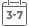# Introduction to Digital Systems: Modeling, Synthesis, and Simulation Using VHDL

• Publish Date: 2011-07-05
• Binding: Hardcover
• Author: Mohammed Ferdjallah
• Sale
• Regular price \$262.86A unique guide to using both modeling and simulation in digital systems design

Digital systems design requires rigorous modeling and simulation analysis that eliminates design risks and potential harm to users. Introduction to Digital Systems: Modeling, Synthesis, and Simulation Using VHDL introduces the application of modeling and synthesis in the effective design of digital systems and explains applicable analytical and computational methods. Through step-by-step explanations and numerous examples, the author equips readers with the tools needed to model, synthesize, and simulate digital principles using Very High Speed Integrated Circuit Hardware Description Language (VHDL) programming.

Extensively classroom-tested to ensure a fluid presentation, this book provides a comprehensive overview of the topic by integrating theoretical principles, discrete mathematical models, computer simulations, and basic methods of analysis. Topical coverage includes:

• Digital systems modeling and simulation

• Integrated logic

• Boolean algebra and logic

• Logic function optimization

• Number systems

• Combinational logic

• VHDL design concepts

• Sequential and synchronous sequential logic

Each chapter begins with learning objectives that outline key concepts that follow, and all discussions conclude with problem sets that allow readers to test their comprehension of the presented material. Throughout the book, VHDL sample codes are used to illustrate circuit design, providing guidance not only on how to learn and master VHDL programming, but also how to model and simulate digital circuits.

Introduction to Digital Systems is an excellent book for courses in modeling and simulation, operations research, engineering, and computer science at the upper-undergraduate and graduate levels. The book also serves as a valuable resource for researchers and practitioners in the fields of operations research, mathematical modeling, simulation, electrical engineering, and computer science.

MORE FROM THIS COLLECTION